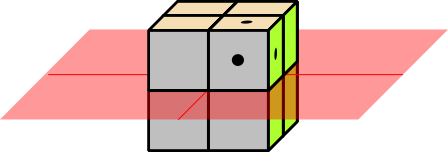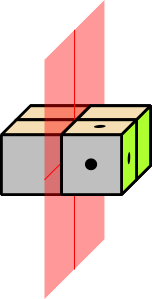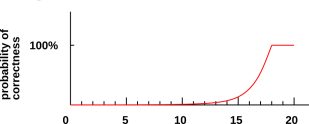[Contents]

Twenty Questions
John Denker

## 1  Overview of the Puzzle

The game of “Twenty Questions” can tell us a lot about what’s learnable and what’s not.

The rules are simple: One player (the oracle) picks a word, any word in the dictionary, and the other player (the analyst) tries to figure out what word it is. The analyst is allowed to ask 20 yes/no questions.

When children play the game, the analyst usually uses the information from previous questions to design the next question. Even so, the analyst often loses the game.

In contrast, an expert analyst never loses. What’s more, the analyst can write down all 20 questions in advance, which means none of the questions can be designed based on the answers to previous questions. This means we have a parallel algorithm, in the sense that the oracle can answer the questions in any order.

I’ll even tell you a set of questions sufficient to achieve this goal:

1) Is the word in the first half of the dictionary?
2) Is the word in the first or third quarter?
3) Is it in an odd-numbered eighth?
*) et cetera.

Each question cuts in half the number of possible words. This can be visualized with the help of figure 1 through figure 4. The cube with the dots represents the right answer. Each yes-no question, if it is properly designed, rules out half of the possibilities. So the effectiveness grows exponentially. A set of N questions can reduce the search-space by a factor of 2N.Figure 1: Eight Possibilities; Rule Out Half of ThemFigure 2: Four Remaining Possibilities; Rule Out Half of ThemFigure 3: Two Remaining Possibilities; Rule Out Half of ThemFigure 4: Only One Remaining Possibility

There are only about 217 words in the largest English dictionaries. So if you use the questions suggested above, after about 17 questions, you know where the word sits on a particular page of the dictionary.

The logic here is the same as in figure 1 through figure 4, except that it doesn’t take place in 3 dimensions, but rather in an abstract 17-dimensional space. Each question reduces the size of the remaining search-space by a factor of 2.

Note that if you ask only 15 questions about a dictionary with 217 entries, you will be able to narrow the search down to a smallish area on the page, but you won’t know which of the words in that area is the right one. You will have to guess, and your guess will be wrong most of the time. The situation is shown in figure 5.Figure 5: Correctness versus Information

You can see that it is important to choose the questions wisely. By way of counterexample, suppose you use non-incisive questions such as

1) Is the word “aardvark”?
2) Is the word “abacus”?
3) Is the word “abaft”?
*) et cetera.

Then each question rules out only one word. This is a linear search, not an exponential search. It would require, on average, hundreds of millions of questions to find the right word. This is not a winning strategy.

### 1.1  Theory

We can formalize and quantify the notion of information. It is complementary to the notion of entropy.

Entropy is a measure of how much we don’t know about the system.

If we are playing 20 questions against an adversary who doesn’t want to help us, we should assume that each word in the dictionary is equally likely to be chosen. This is a minimax assumption. In this case, the entropy is easy to calculate: It is just the logarithm of the number of words. If we choose base-2 logarithms, then the entropy will be denominated in bits. So the entropy of a large English dictionary is about 17 bits.

As we play the game, the answer to each yes/no question gives us one bit of information. We use this to shrink the size of the search space by a factor of 2. That is, the entropy of the part of the dictionary that remains relevant to us goes down by one bit.

In case you were wondering, yes, the entropy that is appears in information theory is exactly the same as the entropy that appears in thermodynamics. Conceptually the same and quantitatively the same. A large physical system usually contains a huge amount of entropy, more than you are likely to find in information-processing applications, but this is only a difference in degree, not a difference in kind ... and there are small physical systems where every last bit of entropy is significant.

### 1.2  Mutations

Consider a modified version of the game: Rather than using an ordinary real dictionary, we use a kooky dictionary, augmented with billion nonsense words.

The base-2 logarithm of a billion is about 30 bits. In this version the 20 questions game is not winnable. After asking 20 questions, there would still be approximately 210 words remaining in the search space. We would have to guess randomly, and we would guess wrong 99.9% of the time.

### 1.3  Implications and Ramifications

This can be seen as an exercise in hypothesis testing, of the sort discussed in reference 1. We have hundreds of millions of hypotheses, and the point is that we cannot consider them one by one. We need a testing strategy that can rule out huge groups of hypotheses at one blow. Another example (on a smaller scale) can be found in reference 2. Additional examples can be found in reference 3 and reference 4.

This has ramifications for data analysis, as discussed in reference 5. It also has ramifications for machine learning, as well as for philosophy, psychology, and pedagogy, as discussed in reference 3; that is, how many examples do you need to see before it is possible to form a generalization? It also has ramifications for the legal system; that is, how much circumstantial evidence constitutes a proof?

One thing we learn is that in some situations a smallish amount of information does you no good at all (you almost always guess the wrong answer), whereas a larger amount can be tremendously powerful (you can find the right answer with confidence). It depends on the amount of data you have, compared to the size of the space you are searching through. If the search space is truly infinite, no amount of data will narrow it down. You need to use some sort of prior knowledge to limit the search space before any sort of induction or learning-from-examples has any hope of working.

This also has ramifications for human learning and thinking. For example, young children are observed to learn language by listening to examples. We know this is so, because in a Chinese-speaking household they learn Chinese, and in a French-speaking household they learn French. The number of examples is large but not infinite. So the question arises, what is there about the structure of the brain that limits the search-space, so as to make learning-from-examples possible? The work of Kohonen sheds some light on this.

## 2  References

1.
John Denker,
“How to Define Hypothesis”
www.av8n.com/physics/hypothesis.htm
2.
John Denker,
“The Twelve-Coins Puzzle”
www.av8n.com/physics/twelve-coins.htm
3.
John Denker,
“Learning, Remembering, and Thinking”
www.av8n.com/physics/thinking.htm
4.
For a general discussion of what entropy is, see:
www.av8n.com/physics/thermo/entropy.html
5.
John Denker
“Data Analysis”
www.av8n.com/physics/data-analysis.htm
[Contents]